GAN-Control: Explicitly Controllable GANs

Amazon One

arXiv [Paper]

# Abstract

We present a framework for training GANs with explicit control over generated images. We are able to control the generated image by settings exact attributes such as age, pose, expression, etc. Most approaches for editing GAN-generated images achieve partial control by leveraging the latent space disentanglement properties, obtained implicitly after standard GAN training. Such methods are able to change the relative intensity of certain attributes, but not explicitly set their values. Recentlyproposed methods, designed for explicit control over human faces, harness morphable 3D face models to allow fine-grained control capabilities in GANs. Unlike these methods, our control is not constrained to morphable 3D face model parameters and is extendable beyond the domain of human faces. Using contrastive learning, we obtain GANs with an explicitly disentangled latent space. This disentanglement is utilized to train control-encoders mapping human-interpretable inputs to suitable latent vectors, thus allowing explicit control. In the domain of human faces we demonstrate control over identity, age, pose, expression, hair color and illumination. We also demonstrate control capabilities of our framework in the domains of painted portraits and dog image generation. We demonstrate that our approach achieves state-of-the-art performance both qualitatively and quantitatively.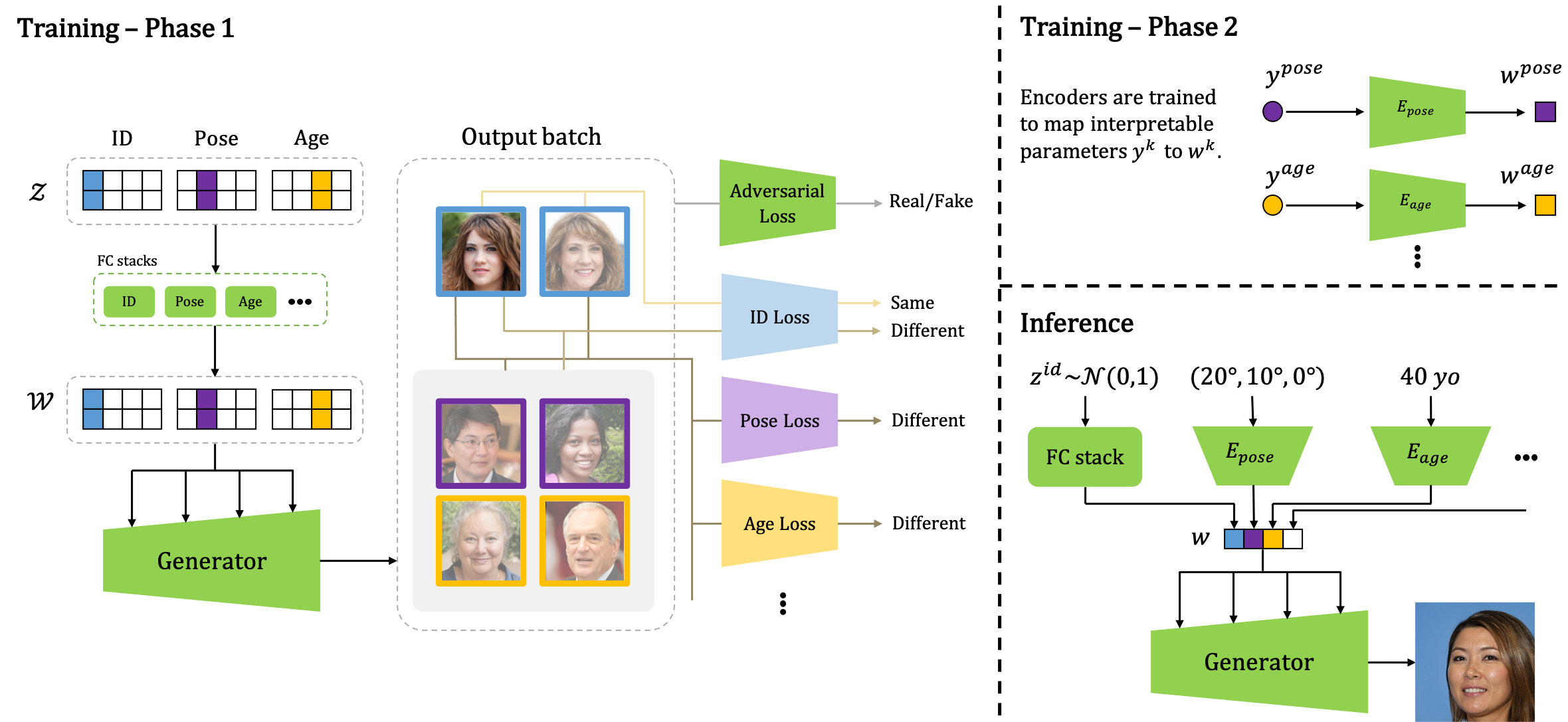Proposed framework: In Phase 1, we construct every batch so that for each attribute, there is a pair of latent vectors sharing a corresponding sub-vector, $$\mathbf{z}^k$$. In addition to the adversarial loss, each image in the batch is compared in a contrastive manner, attribute-by-attribute, to all others, taking into account if it has the same or a different sub-vector. In Phase 2, encoders are trained to map interpretable parameters to suitable latent vectors. Inference: An explicit control over the attribute $$k$$ is achieved by setting the $$k$$th encoder input to a required value.

# Applications

Control over face generation
Explicitly controlling face attributes such as illumination, pose, expression, hair color and age

 Illum. 1 Illum. 2 Illum. 3 Illum. 4 Illum. 5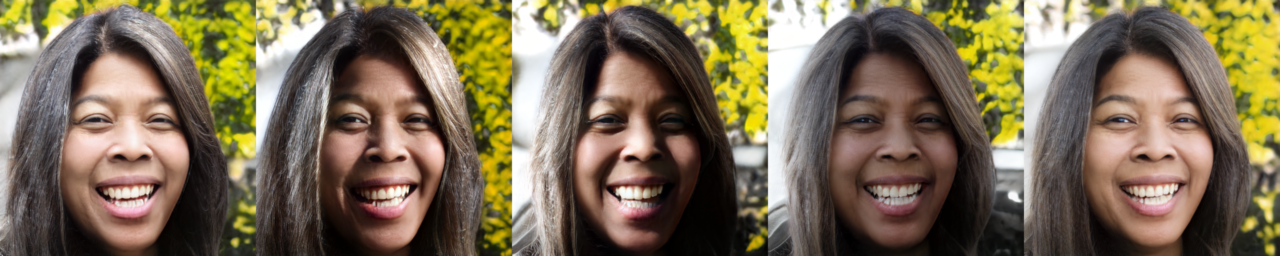Yaw=$$30^\text{o}$$ $$15^\text{o}$$ $$0^\text{o}$$ $$-15^\text{o}$$ $$-30^\text{o}$$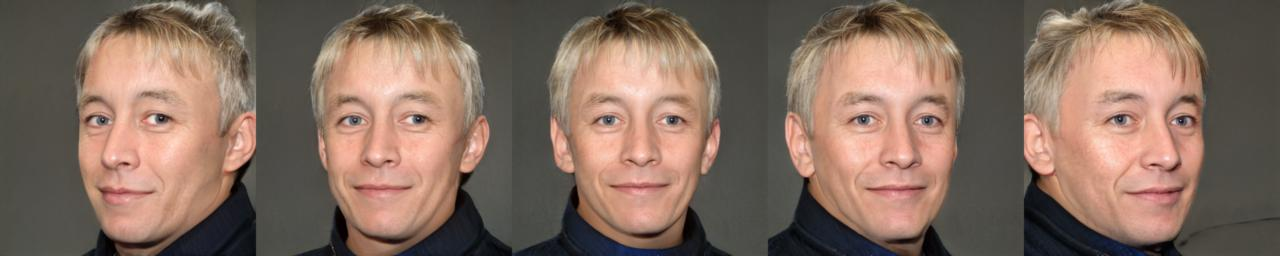Exp. 1 Exp. 2 Exp. 3 Exp. 4 Exp. 5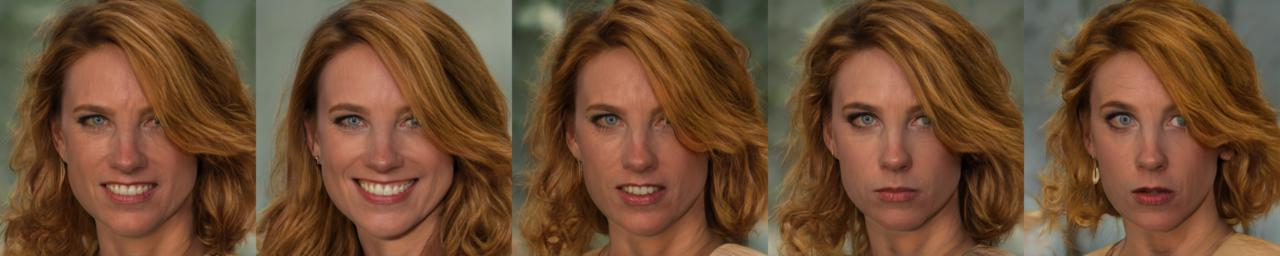Color 1 Color 2 Color 3 Color 4 Color 5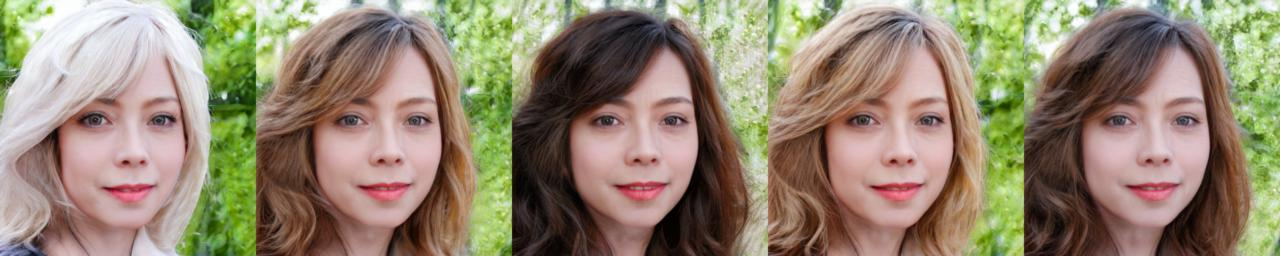Age=$$15$$yo $$30$$yo $$45$$yo $$60$$yo $$75$$yo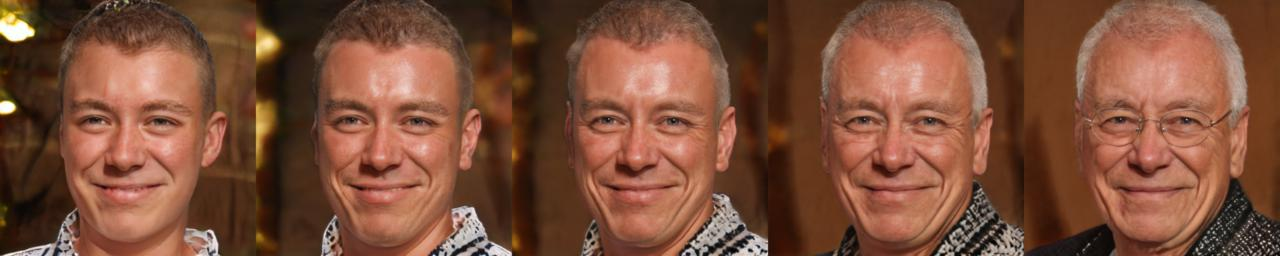Control over painting generation
Explicitly controlling painting attributes such as pose, expression, and age

 Yaw=$$30^\text{o}$$ $$15^\text{o}$$ $$0^\text{o}$$ $$-15^\text{o}$$ $$-30^\text{o}$$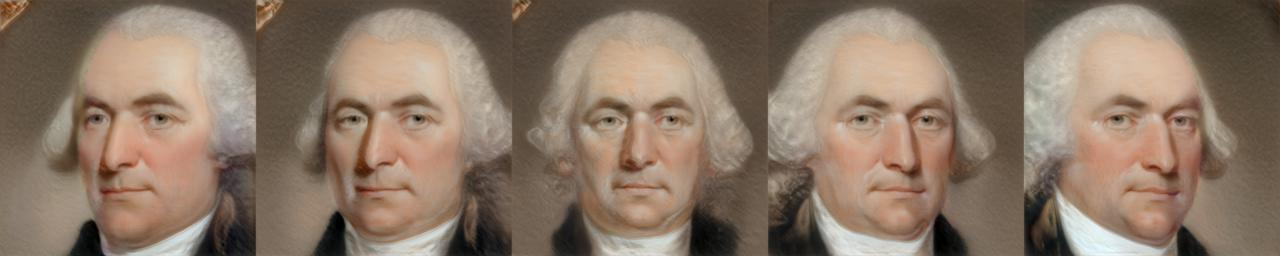Age=$$15$$yo $$30$$yo $$45$$yo $$60$$yo $$75$$yo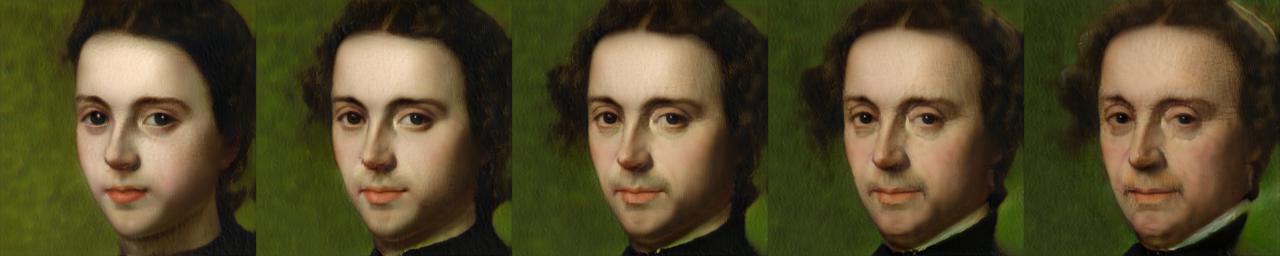Exp. 1 Exp. 2 Exp. 3 Exp. 4 Exp. 5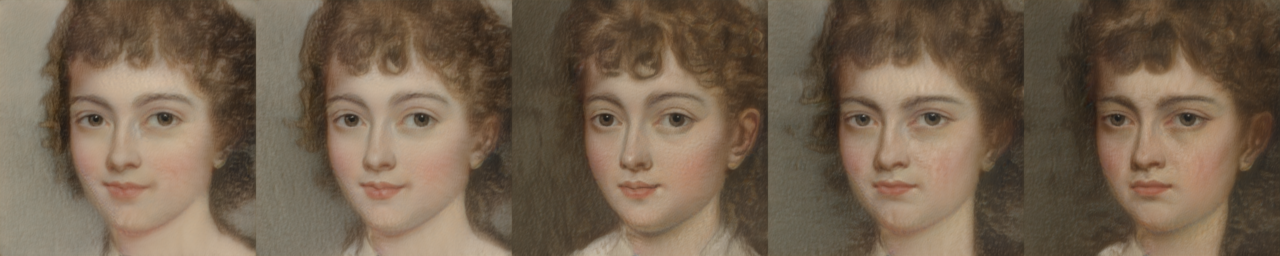Painting artistic style disentanglement
Changing the artistic style of paintings while maintaining all other attributes

 Style. 1 Style. 2 Style. 3 Style. 4 Style. 5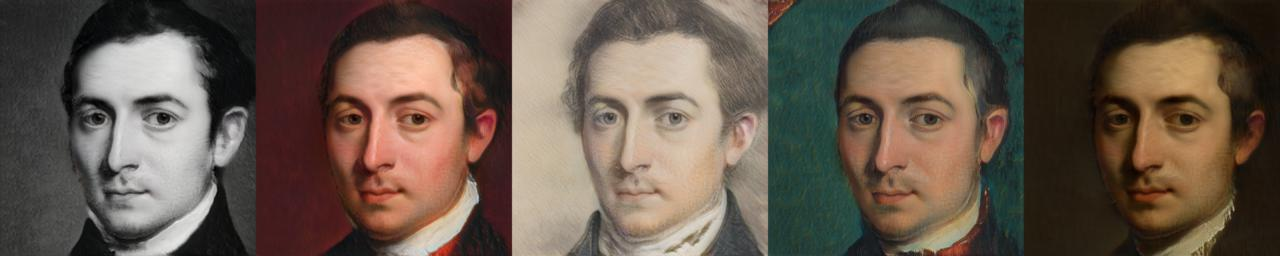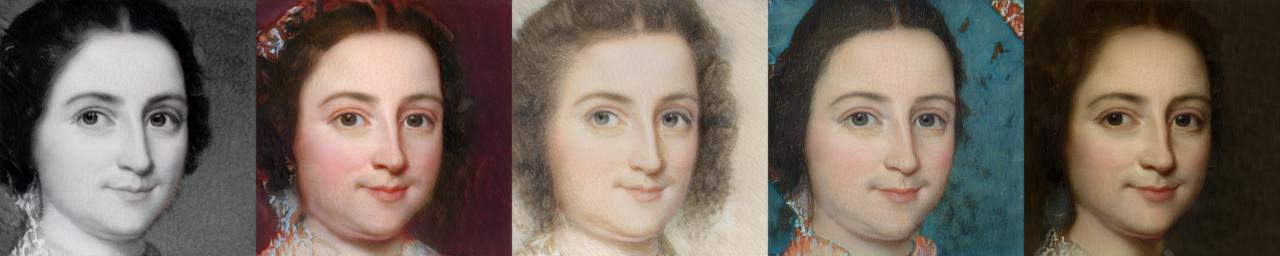Control over dog generation
Explicitly controlling the pose of generated images of dogs

 Yaw=$$30^\text{o}$$ $$15^\text{o}$$ $$0^\text{o}$$ $$-15^\text{o}$$ $$-30^\text{o}$$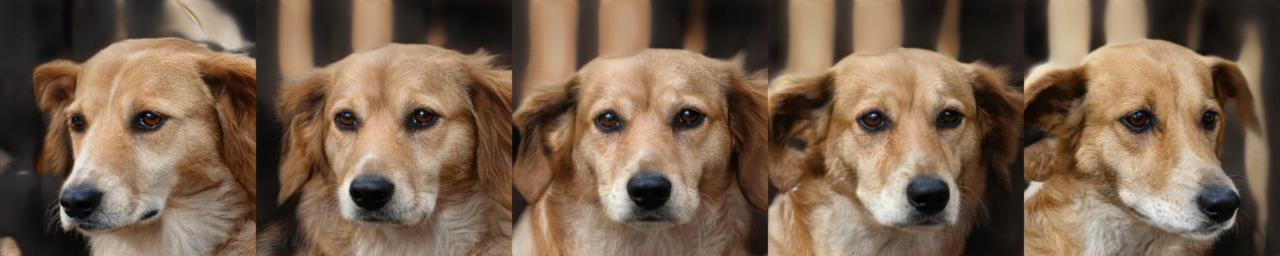Roll=$$20^\text{o}$$ $$-20^\text{o}$$ $$0^\text{o}$$ Pitch=$$-10^\text{o}$$ $$10^\text{o}$$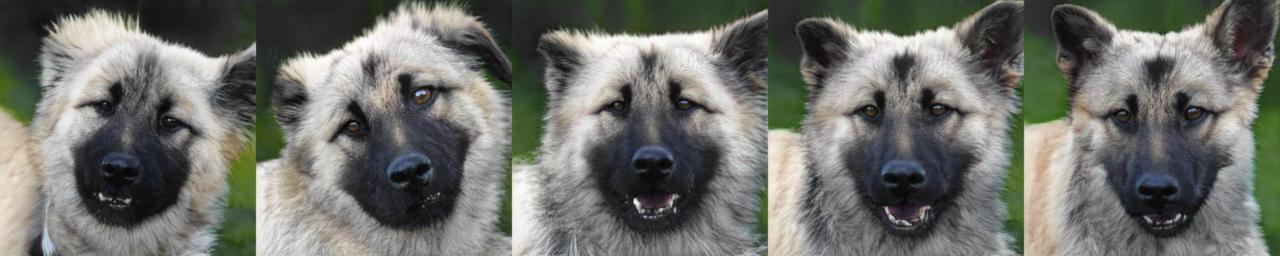Disentangled projection of real images
We propose a new disentangled projection framework to allow real image editing

 Input  Projected Yaw=$$30^\text{o}$$Pitch=$$0^\text{o}$$ Yaw=$$25^\text{o}$$Pitch=$$-15^\text{o}$$ Yaw=$$-25^\text{o}$$Pitch=$$15^\text{o}$$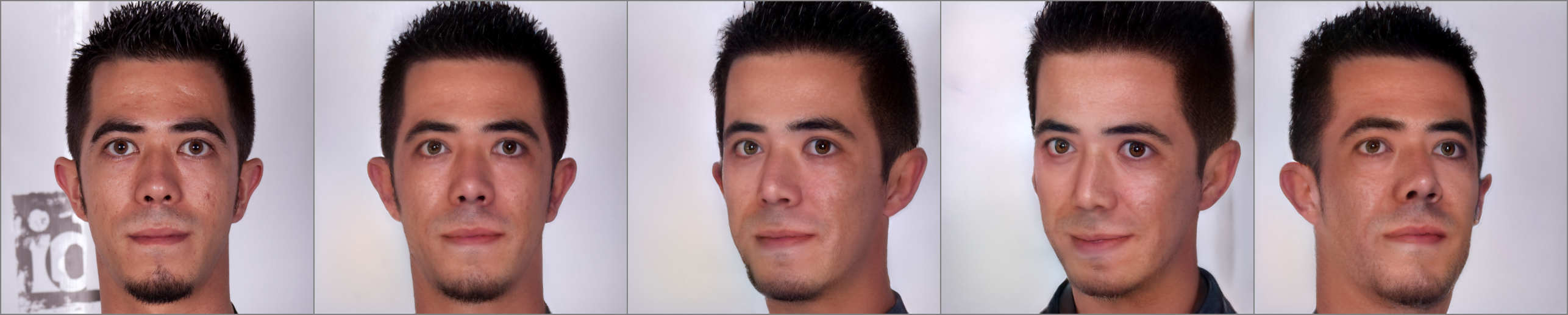Input  Projected Right Front LeftInput  Projected Age=$$15$$yo $$45$$yo $$70$$yo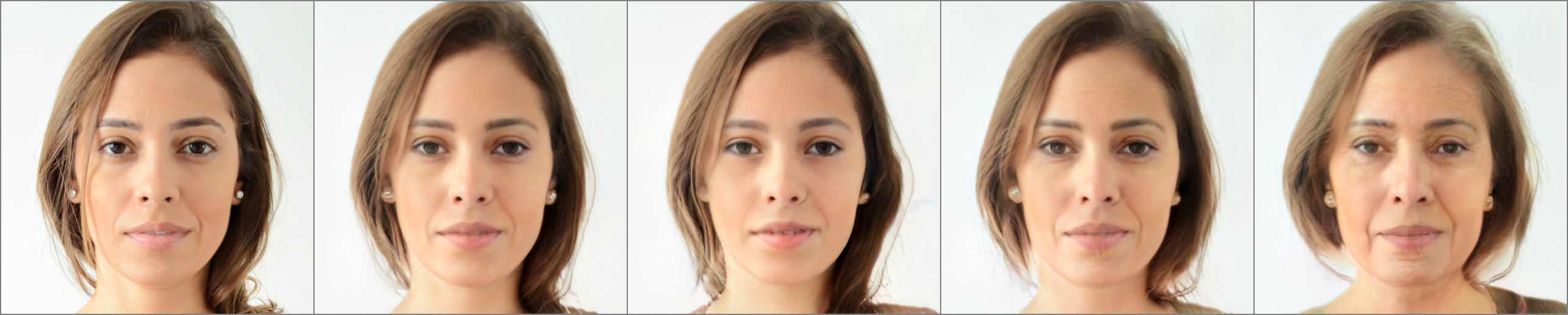Input  Projected Exp. 1 Exp. 2 Exp. 3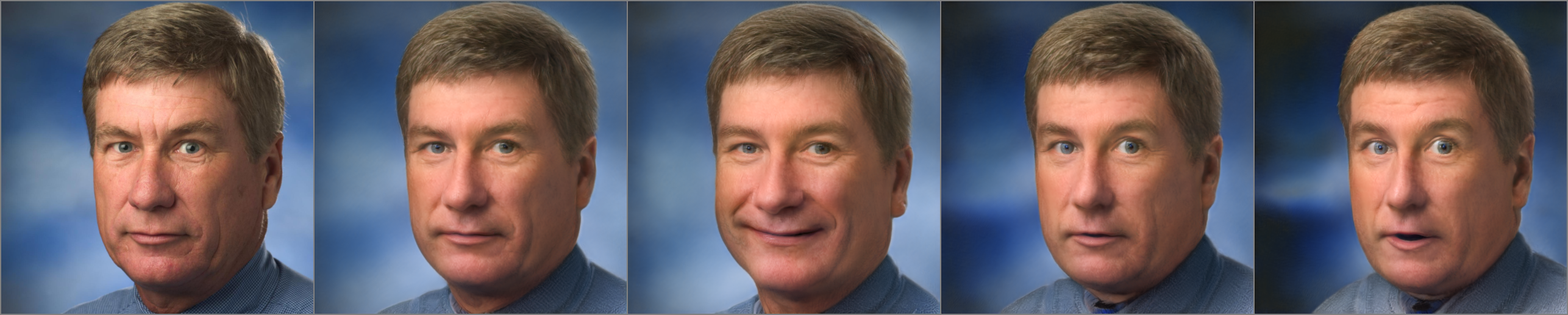# GAN-Control: Explicitly Controllable GANs

Supplementary [pdf]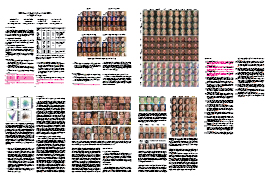# Citation

@misc{shoshan2021gancontrol,
title={GAN-Control: Explicitly Controllable GANs},
author={Alon Shoshan and Nadav Bhonker and Igor Kviatkovsky and Gerard Medioni},
year={2021},
eprint={2101.02477},
archivePrefix={arXiv},
primaryClass={cs.CV}
}